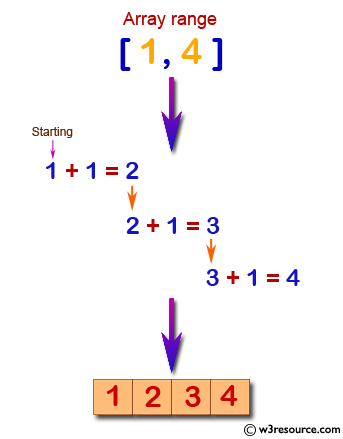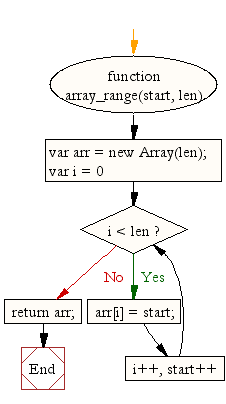# JavaScript: Generate an array of specified length, the content of the array is integer numbers, increase by one from starting

## JavaScript Array: Exercise-40 with Solution

Write a JavaScript function to generate an array of specified length, filled with integer numbers, increase by one from starting position.

Test Data :
console.log(array_range(1, 4));
[1, 2, 3, 4]
console.log(array_range(-6, 4));
[-6, -5, -4, -3]

Pictorial Presentation:Sample Solution:

HTML Code:

``````<!DOCTYPE html>
<html>
<meta charset="utf-8">
<title>JavaScript function to generate an array of given length, the content of the array is integer numbers, increase by one from starting</title>
<body>

</body>
</html>
``````

JavaScript Code:

``````function array_range(start, len)
{
var arr = new Array(len);
for (var i = 0; i < len; i++, start++)
{
arr[i] = start;
}
return arr;
}

console.log(array_range(1, 4));

console.log(array_range(-6, 4));
``````

Sample Output:

```[1,2,3,4]
[-6,-5,-4,-3]
```

Flowchart:ES6 Version:

``````function array_range(start, len)
{
const arr = new Array(len);
for (let i = 0; i < len; i++, start++)
{
arr[i] = start;
}
return arr;
}

console.log(array_range(1, 4));

console.log(array_range(-6, 4));
``````

Live Demo:

Improve this sample solution and post your code through Disqus

What is the difficulty level of this exercise?

Test your Programming skills with w3resource's quiz.

﻿

## JavaScript: Tips of the Day

Array elements

```const list = [1 + 2, 1 * 2, 1 / 2];
console.log(list);
```

Array elements can hold any value. Numbers, strings, objects, other arrays, null, boolean values, undefined, and other expressions such as dates, functions, and calculations.
The element will be equal to the returned value. 1 + 2 returns 3, 1 * 2 returns 2, and 1 / 2 returns 0.5.

Ref: https://bit.ly/3jFRBje